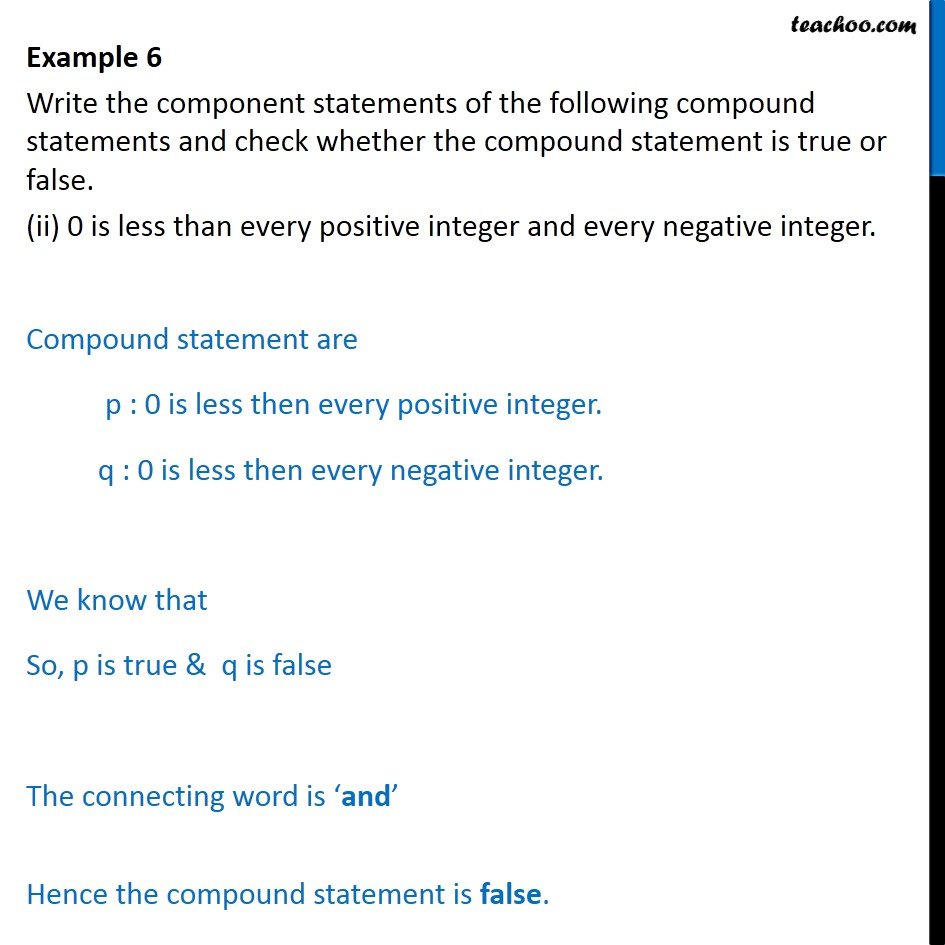Examples

Chapter 14 Class 11 Mathematical Reasoning
Serial order wiseIntroducing your new favourite teacher - Teachoo Black, at only ₹83 per month

### Transcript

Example 6 Write the component statements of the following compound statements and check whether the compound statement is true or false. (ii) 0 is less than every positive integer and every negative integer. Compound statement are p : 0 is less then every positive integer. q : 0 is less then every negative integer. We know that So, p is true & q is false The connecting word is and Hence the compound statement is false.